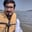Related Tags

python
pandas
series
column
communitycreator

# How to cap outliers from a series/dataframe column in pandasArslan Bajwa

In Python, the pandas library includes built-in functionalities that allow you to perform different tasks with only a few lines of code. One of these functionalities allows you to find and cap outliers from a series or dataframe column.

## Method

In this method, we first initialize a dataframe/series. Then, we set the values of a lower and higher percentile.

We use quantile() to return values at the given quantile within the specified range. Then, we cap the values in series below and above the threshold according to the percentile values.

We replace all of the values of the pandas series in the lower 5th percentile and the values greater than the 95th percentile with respective 5th and 95th percentile values.

#importing pandas and numpy libraries
import pandas as pd
import numpy as np

#initializing pandas series
series = pd.Series(np.logspace(-2, 2, 100))

#set the lower and higher percentile range
lower_percentile = 0.05
higher_percentile = 0.95

#returns values at the given quantile within the specified range
low, high = series.quantile([lower_percentile, higher_percentile])

#cap values below low to low
series[series < low] = low

#cap values above high to high
series[series > high] = high

print(series)

print(lower_percentile, 'low percentile: ', low)
print(higher_percentile, 'high percentile: ', high)

RELATED TAGS

python
pandas
series
column
communitycreator

CONTRIBUTORArslan Bajwa
RELATED COURSES

View all Courses

Keep Exploring

Learn in-demand tech skills in half the time